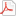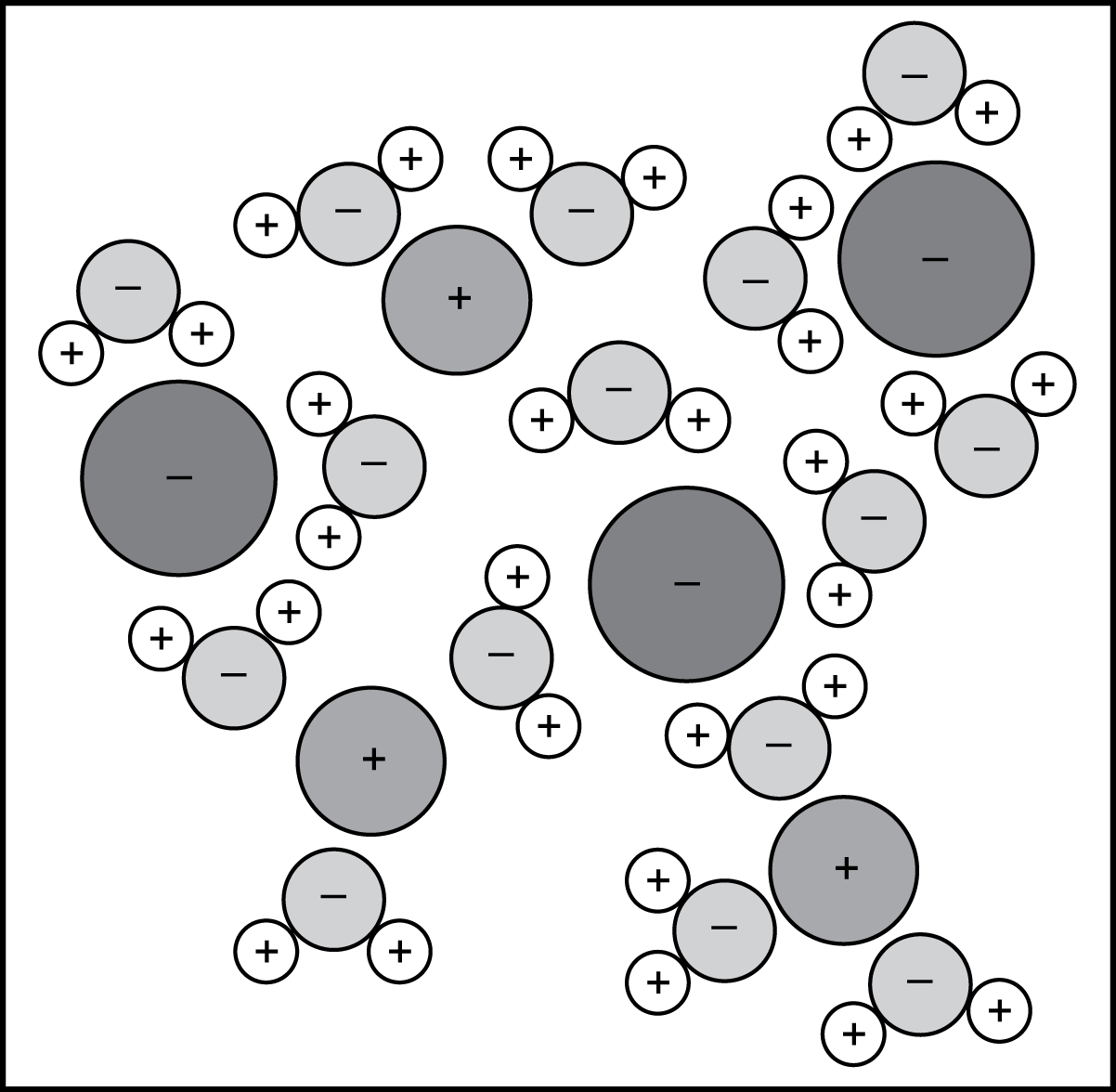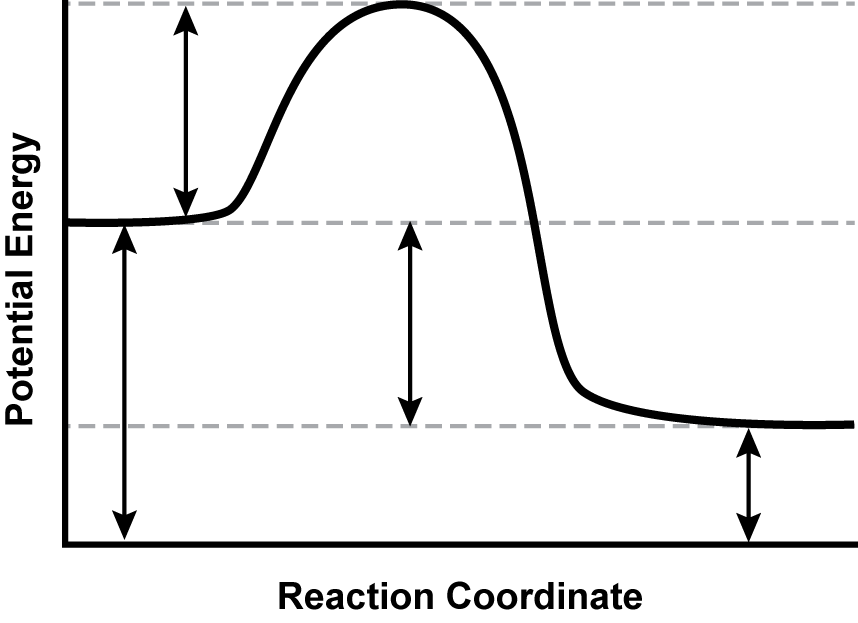# Test Information Guide

## Field 67: Chemistry Sample Multiple-Choice Questions

The following material will be available to you during the test.

Formulas/ConstantsPeriodic Table### Matter and Its Interactions: Periodic Properties

##### Objective 0001Apply knowledge of atomic and subatomic structure and the principles of quantum theory.

1. Use the table below to answer the question that follows.

Isotope Average Atomic Mass (A M U) Abundance (percent)
X 204 2 0 3 point 9 7 3 1 point 4
X 206 2 0 5 point 9 7 4 24 point 1
X 207 2 0 6 point 9 7 6 22 point 1
X 208 2 0 7 point 9 7 7 52 point 4

Element X is composed of four different isotopes. The masses and relative abundances of each isotope are listed in the table. Which of the following values represents the atomic mass of element X?

1. 2 0 6 point 2 2 5 A M U
2. 2 0 7 point 2 1 7 A M U
3. 2 0 7 point 9 7 7 A M U
4. 2 3 2 point 9 1 8 A M U
Correct Response: B.

Correct Response: B.

### Matter and Its Interactions: Chemical Structure and Reactions

##### Objective 0005Apply knowledge of physical and chemical properties and physical and chemical changes.

2. Use the diagram below to answer the question that follows.A minus sign is placed in each of three large circles. A plus sign is placed in each of three medium-sized circles. These are all separated by fifteen smaller circles with minus signs placed inside. Each of these smaller circles is in contact with two very small circles with plus signs placed inside.

Each circle in the diagram represents a single atom. The mixture represented in the diagram is most likely:

1. a saturated mixture of silver nitrate in water.
2. an aqueous solution of sodium chloride.
3. a colloidal dispersion of dust particles.
4. a slurry of iron oxide in water.
Correct Response: B.

Correct Response: B.

##### Objective 0006Apply knowledge of the types and characteristics of chemical reactions.

3. Use the information below to answer the question that follows.

Carbonate Compound Carbonate Molar Mass (grams per molecule) Oxide Compound Oxide Molar Mass (grams per molecule) Ratio of Molar Masses
L I sub 2 C O sub 3 73 point 4 L I sub 2 O 29 point 9 0 point 4 0 7
M G C O sub 3 84 point 3 M G O 40 point 3 0 point 4 7 8
C A C O sub 3 100 point 1 C A O 56 point 1 0 point 5 6 0
M N C O sub 3 114 point 9 M N O 70 point 9 0 point 6 1 7

Metal carbonates decompose into metal oxide and carbon dioxide when heated above the decomposition temperature, for example:

C A C O sub 3 open parens lowercase s close parens plus heat yields C A O open parens lowercase s close parens plus C O open parens lowercase g close parens

In order to determine the identity of an unknown metal carbonate, a sample is heated in a crucible until the mass no longer changes. The sample's mass before and after heating are determined to be 23.14 g and 14.38 g respectively. Based on these results and information in the table, the most likely identity of the unknown metal carbonate is:

1. lithium carbonate (L I sub 2 C O sub 3).
2. magnesium carbonate (M G C O sub 3).
3. calcium carbonate (C A C O sub 3).
4. manganese carbonate (M N C O sub 3).
Correct Response: D.

Correct Response: D.

##### Objective 0007Apply knowledge of the quantitative relationships expressed in chemical equations.

4. Lead(II) nitrate reacts with sodium bromide to produce lead(II) bromide and sodium nitrate according to the following unbalanced reaction.

P B open parens N O sub 3 close parens sub 2 plus N A B R yields P B B R sub 2 plus N A N O sub 3

What is the coefficient for sodium nitrate in the balanced equation?

1. 1
2. 2
3. 3
4. 4
Correct Response: B.

Correct Response: B.

### Matter and Its Interactions: Substances, Mixtures, and Solutions

##### Objective 0008Apply knowledge of the mass relationships in chemical substances.

5. Use the table below to answer the question that follows.

Element Percent of Compound
C 39 point 9 percent
H 6 point 7 percent
O 53 point 4 percent

The table shows the percent composition by mass of a compound. Based on the data, what is the empirical formula of the compound?

1. C H O
2. C H 2 O
3. C sub 2 H sub 2 O sub 2
4. C H sub 3 C O O H
Correct Response: B.

Correct Response: B.

### Motion and Stability: Forces and Interactions

##### Objective 0011Apply knowledge of the connection between molecular structure and forces between particles.

6. Use the table below to answer the question that follows.

Acetic Acid (C H sub 3 C O O H) Carbon Tetrabromide (C B R sub 4) Carbon Tetrachloride (C C L sub 4)
polar solvent nonpolar solid nonpolar solvent
hydrogen bonding London dispersion forces London dispersion forces
colorless liquid white solid colorless liquid
weak acid neither acid nor base neither acid nor base

A student is reviewing the properties of three substances as noted in the table. The student's goal is to determine a reasonable and testable claim about the substances based on the information provided. Which of the following claims is best supported by the information provided in the table?

1. Carbon tetrabromide will dissolve in carbon tetrachloride but will not dissolve in acetic acid.
2. Carbon tetrabromide has longer, stronger bonds than carbon tetrachloride.
3. Acetic acid is a better solvent than carbon tetrachloride due to its polarity.
4. Acetic acid and carbon tetrachloride are miscible liquids.
Correct Response: A.

Correct Response: A.

##### Objective 0012Apply knowledge of the principles of chemical equilibrium.

7. Use the chemical equation below to answer the question that follows.

P B C L sub 2 open parens lowercase S close parens produces P B sup plus 2 open parens lowercase A Q close parens plus 2 C L sup minus open parens lowercase A Q close parens

A saturated lead chloride solution is in equilibrium with the solid salt P B C L sub 2 as shown. Which of the following outcomes will occur if NaCl is added to the solution?

1. The solubility equilibrium will remain unchanged.
2. The solubility equilibrium will shift right.
3. Some P B C L sub 2 will precipitate out of solution.
4. The concentration of P B sup plus 2 open parens lowercase A Q close parens will increase.
Correct Response: C.

Correct Response: C.

### Energy in Chemical Systems

##### Objective 0014Apply knowledge of the principles of thermodynamics and calorimetry.

8. What mass of ice is melted at 0 point 0 degrees Celsius by 1 point 6 7 times 1 0 sup 5 joules of heat?

1. 132 g
2. 199 g
3. 334 g
4. 500 g
Correct Response: D.

Correct Response: D.

##### Objective 0015Apply knowledge of the energy relationships in chemical bonding and chemical reactions.

9. Use the diagram below to answer the question that follows. Apply knowledge of the energy relationships in chemical bonding and chemical reactions.A graph is shown with the y-axis labeled “Potential Energy” and the x-axis labeled “Reaction Coordinate.” A line extends horizontally from the y-axis, two-thirds of the distance up from the origin before increasing, leveling off, falling below its initial y-value, and finally becoming horizontal again.

The potential energy diagram shows the progress of a particular reaction. Which of the following statements about the reaction is best supported by the data in the graph?

1. It requires a catalyst and is endothermic.
2. It needs two activated complexes to go to completion.
3. It requires activation energy and is exothermic.
4. It spontaneously occurs under the conditions represented.
Correct Response: C.

Correct Response: C.

##### Objective 0016Apply knowledge of oxidation-reduction reactions to electrochemistry.

10. A reaction between zinc atoms and silver ions occurs in an electrochemical cell. The standard reduction potential at the anode is minus 0 point 7 6 volts and the standard reduction potential at the cathode is 0 point 8 0 volts. What is the standard cell potential for this electrochemical cell?

1. minus 1 point 6 volts
2. minus 0 point 6 1 volts
3. 0 point 0 4 0 volts
4. 1 point 6 volts